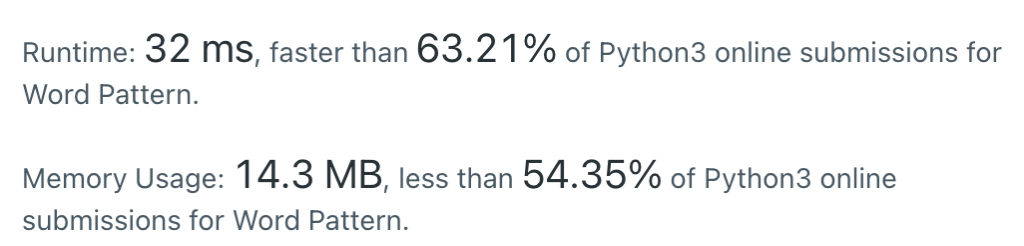#DAY 8
0

## 今日題目：290. Word Pattern（Easy)

Given a pattern and a string s, find if s follows the same pattern.Here follow means a full match, such that there is a bijection between a letter in pattern and a non-empty word in s.

Example 1:
Input: pattern = "abba", s = "dog cat cat dog"
Output: true

Example 2:
Input: pattern = "abba", s = "dog cat cat fish"
Output: false

Example 3:
Input: pattern = "aaaa", s = "dog cat cat dog"
Output: false

### 解題技巧

dictionary 是個好東西：））

### My solution

``````class Solution:
def wordPattern(self, pattern: str, s: str) -> bool:
n = len(pattern)
s = s.split(" ")
temp = {}
if n != len(s):
return False

for i in range(n):
if pattern[i] in temp:
if temp[pattern[i]] != s[i]:
return False
break

else:
if s[i] in temp.values():
return False
break
else:
temp[pattern[i]] = s[i]
return True

``````

### Result